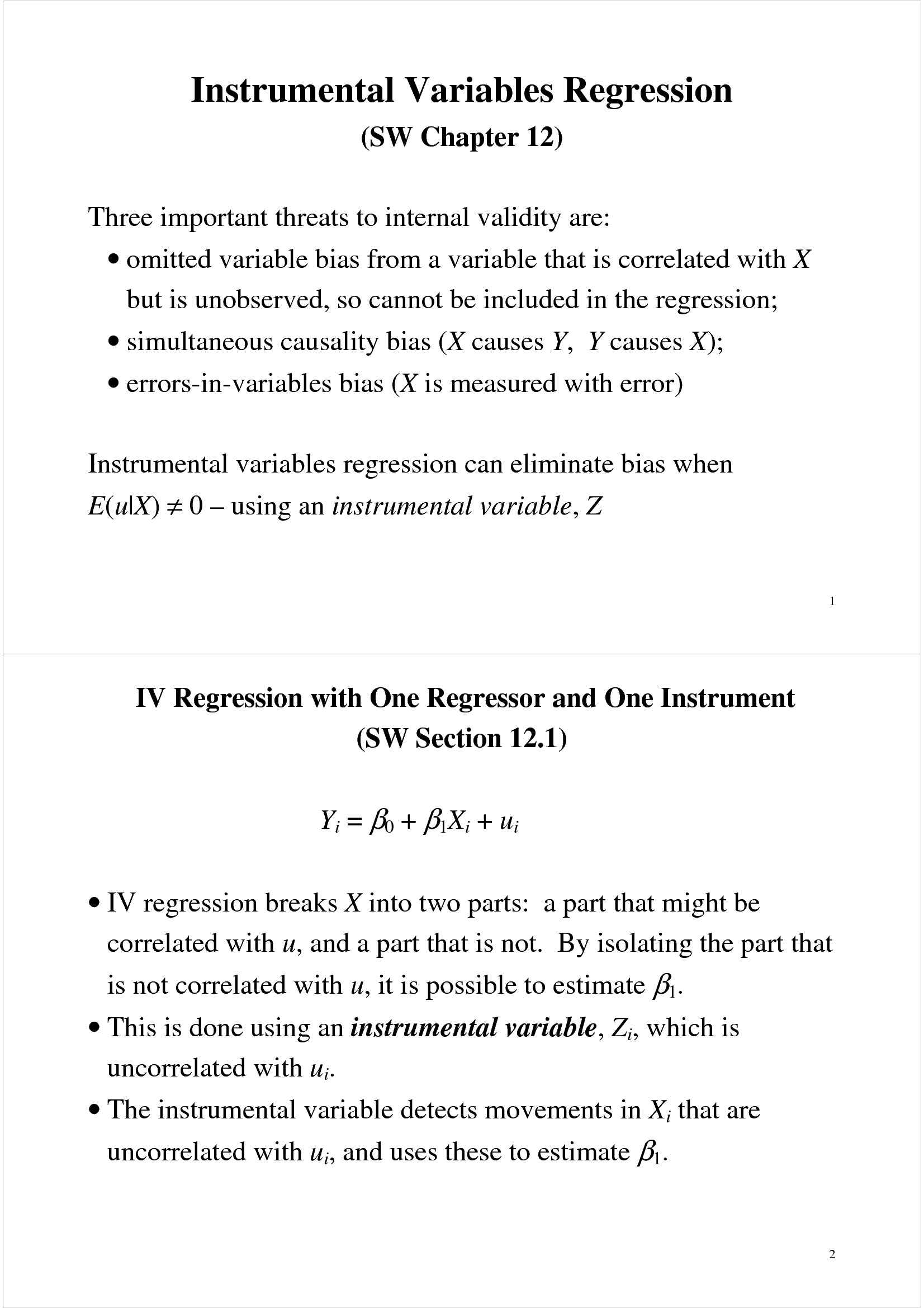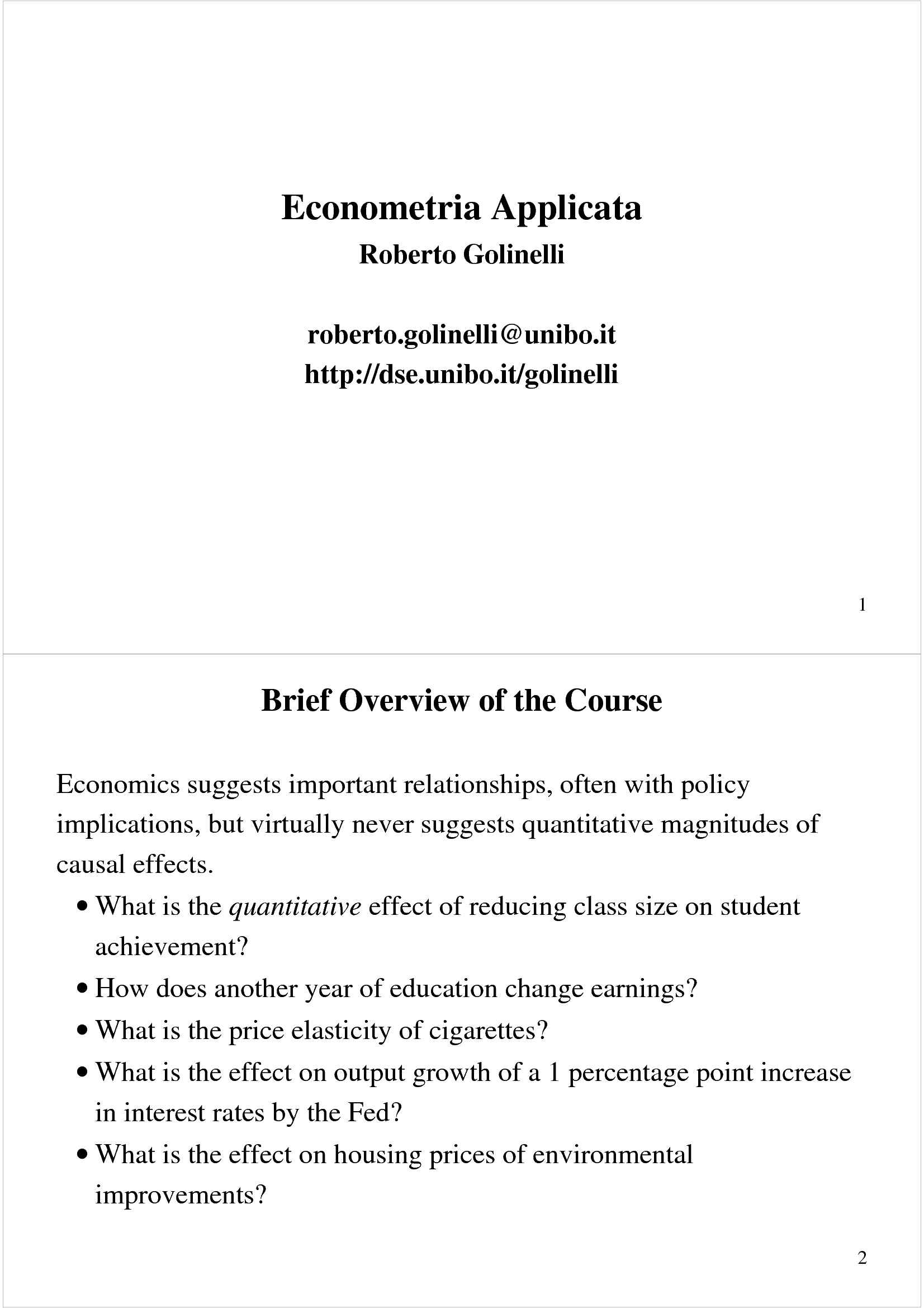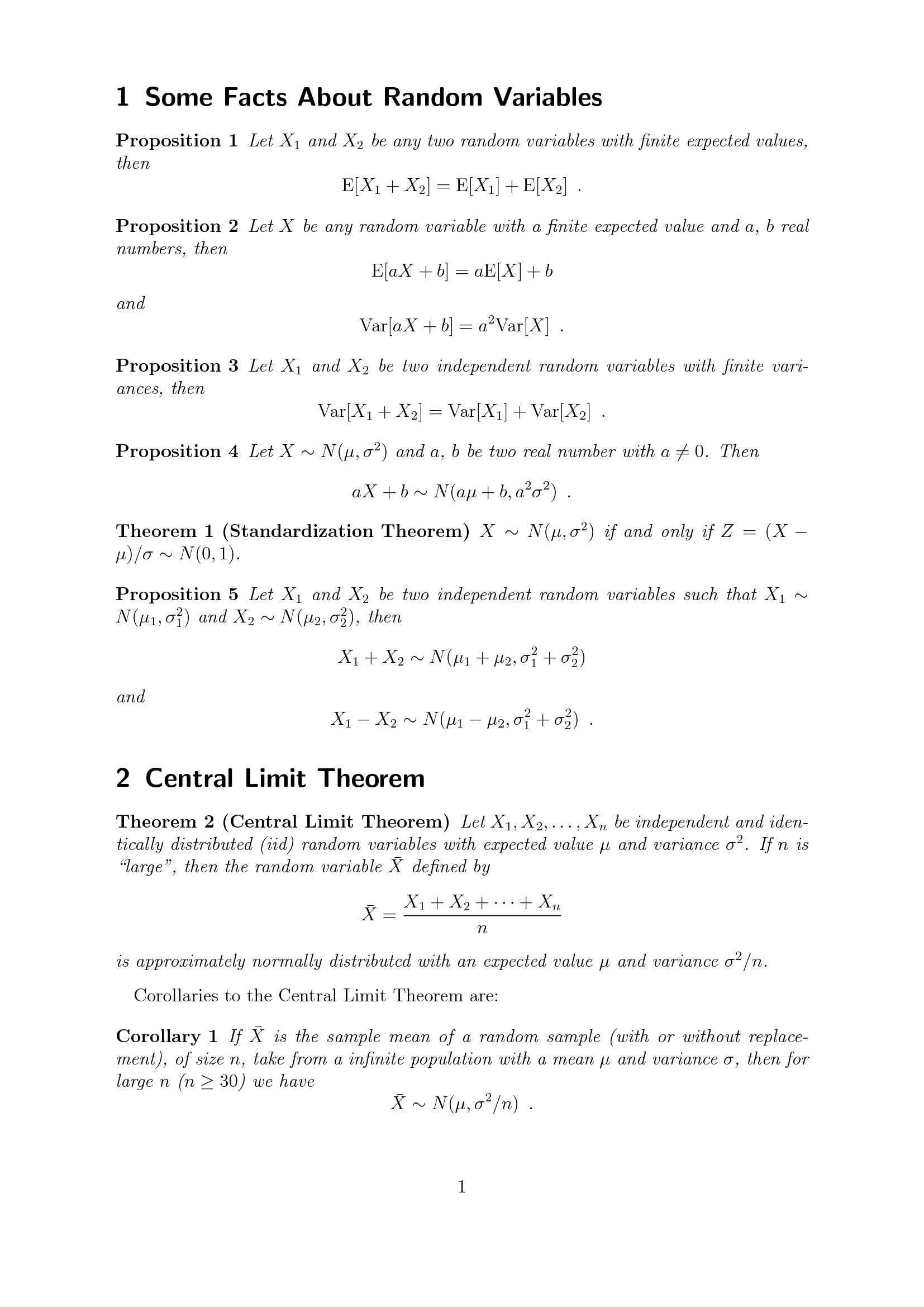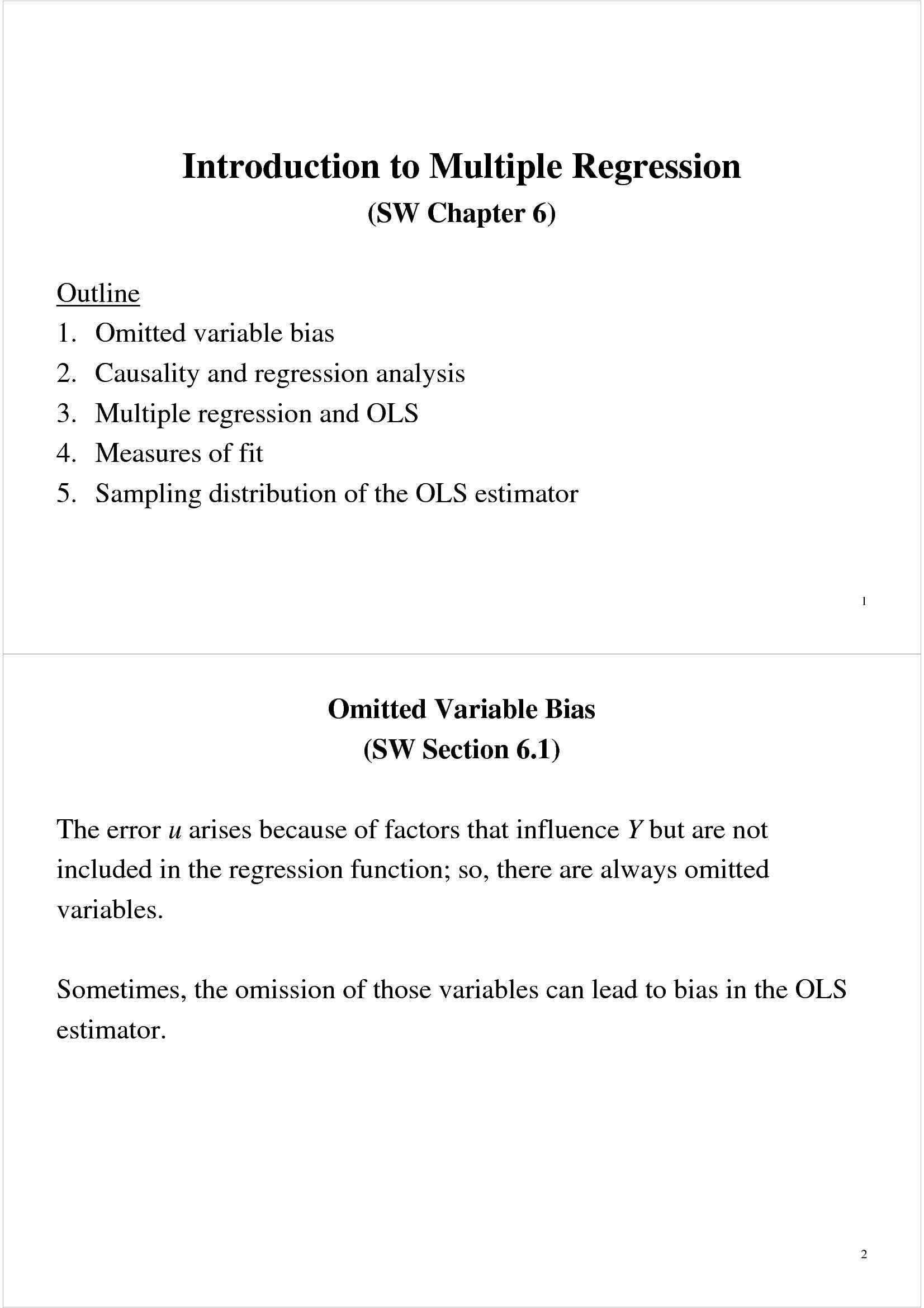Che materia stai cercando?

# Regressione Forecasting e Time Series

Materiale didattico per il corso di Econometria applicata del prof. Roberto Golinelli. Trattasi di slides in lingua inglese a cura del docente, all'interno delle quali sono affrontati i seguenti argomenti: il modello di regressione Forecasting e Time Series; autocorrelazione ed autocovarianza; il modello... Vedi di più

Esame di Econometria applicata docente Prof. R. Golinelli

Anteprima

### ESTRATTO DOCUMENTO

Other economic time series, ctd: 17

Stationarity: a key requirement for external validity of time series

regression

Stationarity says that history is relevant:

For now, assume that Y is stationary (we return to this later).

t 18

Autoregressions (SW Section 14.3)

A natural starting point for a forecasting model is to use past values of Y

, Y ,…) to forecast Y .

(that is, Y t–1 t–2 t

• An autoregression is a regression model in which Y is regressed

t

against its own lagged values.

• The number of lags used as regressors is called the order of the

autoregression. is regressed against Y

- In a first order autoregression, Y t t–1

th

- In a p order autoregression, Y is regressed against Y ,Y ,…,Y .

t t–1 t–2 t–p 19

20

The First Order Autoregressive (AR(1)) Model

The population AR(1) model is

β β

= + Y + u

Y t 0 1 t–1 t

β β

• and do not have causal interpretations

0 1

β

• if = 0, Y is not useful for forecasting Y

1 t–1 t

• The AR(1) model can be estimated by OLS regression of Y against Y

t t–

1 β β ≠

• = 0 v. 0 provides a test of the hypothesis that Y is

Testing 1 1 t–1

not useful for forecasting Y t 21

Example: AR(1) model of the change in inflation

Estimated using data from 1962:I – 2004:IV:

∆ ∆Inf

= 2

I n̂ f 0.017 – 0.238 = 0.05

## R

t–1

t (0.126) (0.096)

Is the lagged change in inflation a useful predictor of the current change

in inflation?

• t = –.238/.096 = –2.47 > 1.96 (in absolute value)

β

• → : = 0 at the 5% significance level

Reject H 0 1

Yes, the lagged change in inflation is a useful predictor of current change

2

in inflation–but the is pretty low!

R 22

Forecasts: terminology and notation

• Predicted values are “in-sample” (the usual definition)

• Forecasts are “out-of-sample” – in the future

• Notation:

= forecast of Y based on Y ,Y ,…, using the population

## Y

o T+1|T T+1 T T–1

(true unknown) coefficients

ˆ = forecast of Y based on Y ,Y ,…, using the estimated

o + T+1 T T–1

T 1|

## T

coefficients, which are estimated using data through period T.

For an AR(1):

o β β

Y = + Y

T+1|T 0 1 T

β β β β

ˆ ˆ ˆ ˆ

ˆ

= + Y , where and are estimated using data

Y + T

T 1|

T 0 1 0 1

through period T. 23

Forecast errors

The one-period ahead forecast error is,

ˆ

forecast error = Y Y +

T+1 T 1|

## T

The distinction between a forecast error and a residual is the same as

between a forecast and a predicted value:

• a residual is “in-sample”

• a forecast error is “out-of-sample” – the value of Y isn’t used in the

T+1

estimation of the regression coefficients 24

Example: forecasting inflation using an AR(1)

AR(1) estimated using data from 1962:I – 2004:IV:

∆ ∆Inf

=

I n̂ f 0.017 – 0.238 t–1

t

Inf = 1.6 (units are percent, at an annual rate)

2004:III

Inf = 3.5

2004:IV

∆Inf = 3.5 – 1.6 = 1.9

2004:IV ∆Inf is:

The forecast of 2005:I

∆ =

I n̂ f 0.017 – 0.238×1.9 = -0.44

2005 : I | 2004 : IV

so ∆

= =

I n̂ f I n̂ f

Inf + = 3.5 – 0.4 = 3.1%

2005 : I | 2004 : IV 2005 : I | 2004 : IV

2004:IV 25

The AR(p) model: using multiple lags for forecasting

th order autoregressive model (AR(p)) is

The p β β β β

= + Y + Y + … + Y + u

Y t 0 1 t–1 2 t–2 p t–p t

• The AR(p) model uses p lags of Y as regressors

• The AR(1) model is a special case

• The coefficients do not have a causal interpretation

• ,…,Y do not further help forecast Y ,

To test the hypothesis that Y t–2 t–p t

beyond Y , use an F-test

t–1

• Use t- or F-tests to determine the lag order p

• Or, better, determine p using an “information criterion” (more on this

later…) 26

Example: AR(4) model of inflation

∆ =

I n̂ f .02 – .26∆Inf – .32∆Inf + .16∆Inf – .03∆Inf ,

t–1 t–2 t–3 t–4

t (.12) (.09) (.08) (.08) (.09)

2 = 0.18

## R

• F-statistic testing lags 2, 3, 4 is 6.91 (p-value < .001)

• 2 increased from .05 to .18 by adding lags 2, 3, 4

## R

• So, lags 2, 3, 4 (jointly) help to predict the change in inflation, above

and beyond the first lag – both in a statistical sense (are statistically 2 )

significant) and in a substantive sense (substantial increase in the R 27

∆Inf,

Digression: we used not Inf, in the AR’s. Why?

The AR(1) model of Inf is an AR(2) model of Inf :

t–1 t

β β ∆Inf

∆Inf = + + u

t 0 1 t–1 t

or β β

– Inf = + (Inf – Inf ) + u

Inf

t t–1 0 1 t–1 t–2 t

or β β β

Inf = Inf + + Inf – Inf + u

t t–1 0 1 t–1 1 t–2 t

β β β

+ (1+ )Inf – Inf + u

= 0 1 t–1 1 t–2 t 28

∆Inf

So why use , not Inf ?

t t β β ∆Inf

∆Inf: ∆Inf = + + u

AR(1) model of t 0 1 t–1 t

γ γ γ

AR(2) model of Inf: Inf = + Inf + Inf + v

t 0 1 t 2 t–1 t

• When Y is strongly serially correlated, the OLS estimator of the AR

t

coefficient is biased towards zero.

• In the extreme case that the AR coefficient = 1, Y isn’t stationary: the

t

u ’s accumulate and Y blows up.

t t

• If Y isn’t stationary, our regression theory are working with here breaks

t

down

• Here, Inf is strongly serially correlated – so to keep ourselves in a

t ∆Inf

framework we understand, the regressions are specified using

• More on this later… 29

Time Series Regression with Additional Predictors and the

(SW Section 14.4)

• So far we have considered forecasting models that use only past values

of Y

• It makes sense to add other variables (X) that might be useful predictors

of Y, above and beyond the predictive value of lagged values of Y:

β β β δ δ

= + Y + … + Y + X + … + X + u

Y t 0 1 t–1 p t–p 1 t–1 r t–r t

• This is an autoregressive distributed lag model with p lags of Y and r

lags of X … ADL(p,r). 30

31

Example: inflation and unemployment

According to the “Phillips curve,” if unemployment is above its

equilibrium, or “natural,” rate, then the rate of inflation will increase.

∆Inf

That is, is related to lagged values of the unemployment rate, with a

t

negative coefficient

• The rate of unemployment at which inflation neither increases nor

decreases is often called the “non-accelerating rate of inflation”

unemployment rate (the NAIRU).

• Is the Phillips curve found in US economic data?

• Can it be exploited for forecasting inflation?

• Has the U.S. Phillips curve been stable over time? 32

The empirical U.S. “Phillips Curve,” 1962 – 2004 (annual)

∆Inf

One definition of the NAIRU is that it is the value of u for which = 0

– the x intercept of the regression line. 33

The empirical (backwards-looking) Phillips Curve, ctd.

ADL(4,4) model of inflation (1962 – 2004):

∆ =

I n̂ f 1.30 – .42∆Inf – .37∆Inf + .06∆Inf – .04∆Inf

t–1 t–2 t–3 t–4

t (.44) (.08) (.09) (.08) (.08)

+ 3.04Unem – 0.38Unem + .25Unemp

– 2.64Unem t–1 t–2 t–3 t–4

(.46) (.86) (.89) (.45)

• 2 2

= 0.34 – a big improvement over the AR(4), for which = .18

R R 34

The test of the joint hypothesis that none of the X’s is a useful predictor,

above and beyond lagged values of Y, is called a Granger causality test

“causality” is an unfortunate term here: Granger Causality simply refers

to (marginal) predictive content. 35

Forecast uncertainty and forecast intervals

Why do you need a measure of forecast uncertainty?

• To construct forecast intervals

• To let users of your forecast (including yourself) know what degree of

accuracy to expect

Consider the forecast β β β

ˆ ˆ ˆ

ˆ = Y + X

Y +

+ T T

T 1|

T 0 1 1

The forecast error is: β β β

β β β

ˆ ˆ ˆ

ˆ –

– = u – [( ) + ( – )Y + ( – )X ]

Y Y +

T+1 T+1 0 1 T 2 T

## T T

1| 0 1 1 36

The mean squared forecast error (MSFE) is,

ˆ 2 2

E(Y – ) = E(u ) +

Y +

T+1 T+1

T 1|

T β β β

β β β

ˆ ˆ ˆ 2

) + ( – )Y + ( – )X ]

+ E[( 0 1 T 2 T

0 1 1

• ) + uncertainty arising because of estimation error

MSFE = var(u T+1

• If the sample size is large, the part from the estimation error is (much)

smaller than var(u ), in which case

T+1 )

MSFE var(u T+1

• The root mean squared forecast error (RMSFE) is the square root of

the MS forecast error: − ˆ 2

RMSFE = E [(

Y Y ) ]

+ +

T 1 T 1|

T 37

The root mean squared forecast error (RMSFE)

− ˆ 2

RMSFE = E [(

Y Y ) ]

+ +

T 1 T 1|

## T

• The RMSFE is a measure of the spread of the forecast error

distribution.

• The RMSFE is like the standard deviation of u , except that it

t

explicitly focuses on the forecast error using estimated coefficients,

not using the population regression line.

• The RMSFE is a measure of the magnitude of a typical forecasting

“mistake” 38

Three ways to estimate the RMSFE σ

≈ , so estimate the RMSFE by the

1. Use the approximation RMSFE u

## SER.

2. Use an actual forecast history for t = t ,…, T, then estimate by

1

T 1

1 ∑

= − ˆ 2

## M

Ŝ FE (

Y Y )

+ +

− + t 1 t 1|

t

T t 1 = −

t t 1

1 1

Usually, this isn’t practical – it requires having an historical record

of actual forecasts from your model

forecast history, that is, simulate the forecasts you

3. Use a simulated

2, with these pseudo out-of-sample forecasts… 39

The method of pseudo out-of-sample forecasting

• –1,…,T–1

Re-estimate your model every period, t = t

1

• Compute your “forecast” for date t+1 using the model estimated

through t

• Compute your pseudo out-of-sample forecast at date t, using the

ˆ .

model estimated through t–1. This is Y +

t 1|

t

• ˆ

Compute the poos forecast error, Y – Y +

t+1 t 1|

t

• Plug this forecast error into the MSFE formula,

T 1

1 ∑

= − ˆ 2

## M

Ŝ FE (

Y Y )

+ +

− + t 1 t 1|

t

T t 1 = −

t t 1

1 1

Why the term “pseudo out-of-sample forecasts”? 40

Using the RMSFE to construct forecast intervals

If u is normally distributed, then a 95% forecast interval can be

T+1

constructed as

ˆ RM

## Ŝ FE

± 1.96×

Y −

T |

T 1

Note: isn’t a

1. A 95% forecast interval is not a confidence interval (Y T+1

nonrandom coefficient, it is random!)

2. This interval is only valid if u is normal – but still might be a

T+1

reasonable approximation and is a commonly used measure of

forecast uncertainty ± RM

## Ŝ FE

3. Often “67%” forecast intervals are used: 1.00 × 41

42

Example #1: the Bank of England “Fan Chart (rivers of blood)”

http://www.bankofengland.co.uk/publications/inflationreport/index.htm 43

Example #2: Monthly Bulletin of the European Central Bank, Dec.

2005, Staff macroeconomic projections

Precisely how, did they compute these intervals?

http://www.ecb.int/pub/mb/html/index.en.html 44

Example #3: Fed, Semiannual Report to Congress, 7/04

Economic projections for 2004 and 2005

Federal Reserve Governors

and Reserve Bank presidents

Indicator Range Central tendency

2005

Change, fourth quarter

to fourth quarter

Nominal GDP 4-3/4 to 6-1/2 5-1/4 to 6

Real GDP 3-1/2 to 4 3-1/2 to 4

PCE price index excl food and energy 1-1/2 to 2-1/2 1-1/2 to 2

Average level, fourth quarter

Civilian unemployment rate 5 to 5-1/2 5 to 5-1/4

How did they compute these intervals?

http://www.federalreserve.gov/boarddocs/hh/ 45

Lag Length Selection Using Information Criteria

(SW Section 14.5)

How to choose the number of lags p in an AR(p)?

• Omitted variable bias is irrelevant for forecasting

• You can use sequential “downward” t- or F-tests; but the models

chosen tend to be “too large” (why?)

• Another – better – way to determine lag lengths is to use an

information criterion

• Information criteria trade off bias (too few lags) vs. variance (too

many lags)

• Two IC are the Bayes (BIC) and Akaike (AIC)… 46

The Bayes Information Criterion (BIC)

 

SSR ( p ) ln T

+ +

BIC(p) = ln ( p 1)

 

 

## T T

• First term: always decreasing in p (larger p, better fit)

• Second term: always increasing in p.

The variance of the forecast due to estimation error increases with p

o – so you don’t want a forecasting model with too many coefficients

– but what is “too many”?

This term is a “penalty” for using more parameters – and thus

o increasing the forecast variance.

• Minimizing BIC(p) trades off bias and variance to determine a “best”

value of p for your forecast.

p

## BIC

ˆ

The result is that p! (SW, App. 14.5)

p

o 47

Another information criterion: Akaike Information Criterion (AIC)

 

SSR ( p ) 2

+ +

AIC(p) = p

ln ( 1)

 

 

## T T

 

SSR ( p ) ln T

+ +

BIC(p) = ln ( p 1)

 

 

## T T

The penalty term is smaller for AIC than BIC (2 < lnT)

AIC estimates more lags (larger p) than the BIC

o This might be desirable if you think longer lags might be

o important.

However, the AIC estimator of p isn’t consistent – it can

o overestimate p – the penalty isn’t big enough 48

Example: AR model of inflation, lags 0 – 6: 2

# Lags BIC AIC R

0 1.095 1.076 0.000

1 1.067 1.030 0.056

2 0.900 0.181

0.955

3 0.957 0.203

0.884

4 0.986 0.895 0.204

5 1.016 0.906 0.204

6 1.046 0.918 0.204

• BIC chooses 2 lags, AIC chooses 3 lags.

• 2

If you used the R to enough digits, you would (always) select the

largest possible number of lags. 49

Generalization of BIC to multivariate (ADL) models

Let K = the total number of coefficients in the model (intercept, lags of Y,

lags of X). The BIC is,

 

SSR ( K ) ln T

+

BIC(K) = ln K

 

 

## T T

• Can compute this over all possible combinations of lags of Y and lags

of X (but this is a lot)!

• In practice you might choose lags of Y by BIC, and decide whether or

not to include X using a Granger causality test with a fixed number of

lags (number depends on the data and application) 50

Nonstationarity I: Trends

(SW Section 14.6)

• So far, we have assumed that the data are well-behaved – technically,

that the data are stationary.

• Now we will discuss two of the most important ways that, in practice,

data can be nonstationary (that is, deviate from stationarity). You

need to be able to recognize/detect nonstationarity, and to deal with it

when it occurs.

• Two important types of nonstationarity are:

Trends (SW Section 14.6)

o Structural breaks (model instability) (SW Section 14.7)

o

• Up now: trends 51

Outline of discussion of trends in time series data:

1. What is a trend?

2. What problems are caused by trends?

3. How do you detect trends (statistical tests)?

4. How to address/mitigate problems raised by trends 52

PAGINE

42

PESO

6.57 MB

AUTORE

PUBBLICATO

+1 anno fa

### DESCRIZIONE DISPENSA

Materiale didattico per il corso di Econometria applicata del prof. Roberto Golinelli. Trattasi di slides in lingua inglese a cura del docente, all'interno delle quali sono affrontati i seguenti argomenti: il modello di regressione Forecasting e Time Series; autocorrelazione ed autocovarianza; il modello di autoregressione con ritardo; il test della casualità di Granger; il Bayes Information Criterion (BIC).

DETTAGLI
Corso di laurea: Corso di laurea in economia, mercati e istituzioni
SSD:
Università: Bologna - Unibo
A.A.: 2011-2012

I contenuti di questa pagina costituiscono rielaborazioni personali del Publisher Atreyu di informazioni apprese con la frequenza delle lezioni di Econometria applicata e studio autonomo di eventuali libri di riferimento in preparazione dell'esame finale o della tesi. Non devono intendersi come materiale ufficiale dell'università Bologna - Unibo o del prof Golinelli Roberto.

Acquista con carta o conto PayPal

Scarica il file tutte le volte che vuoi

Paga con un conto PayPal per usufruire della garanzia Soddisfatto o rimborsato

RecensioniTi è piaciuto questo appunto? Valutalo!

## Altri appunti di Econometria applicataDispensa

### Regressione con variabili strumentaliDispensa

### Econometria - elementiDispensa

### Riepilogo di concetti statisticiDispensa